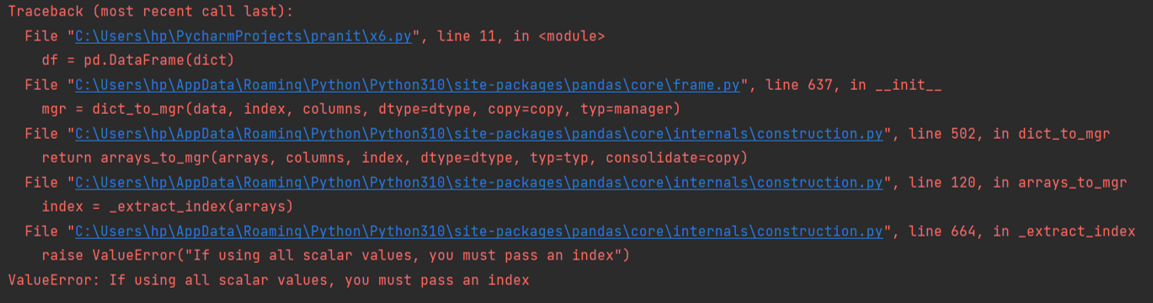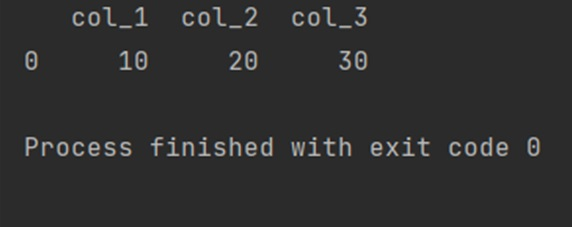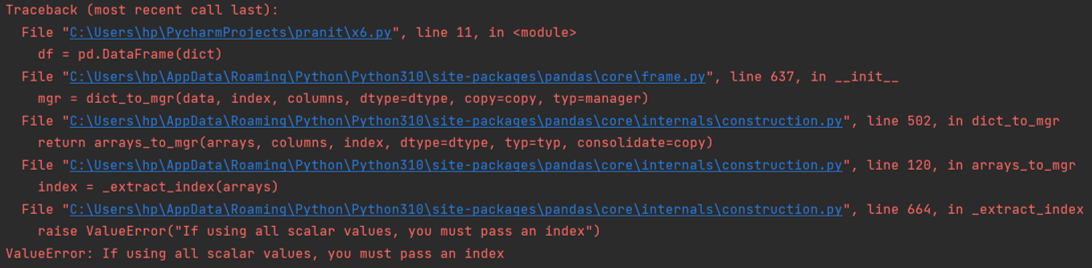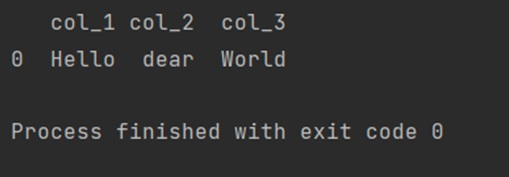# ValueError: If using all scalar values, you must pass an index, How to Fix it?

Solution for Constructing pandas DataFrame from values in variables gives "ValueError: If using all scalar values, you must pass an index". By Pranit Sharma Last updated : April 19, 2023

## Why "ValueError: If using all scalar values, you must pass an index" Occurs?

We can create a DataFrame with the help of a dictionary but when we use scaler values or even strings as a value inside the dictionary, pandas will raise an error.

Consider the following examples – It will generate ValueError: If using all scalar values, you must pass an index.

### Example

```# Importing pandas package

import pandas as pd

# Creating a Dictionary and setting integers as values

dict = {'col_1':10,'col_2':20,'col_3':30}

# Creating a DataFrame

df = pd.DataFrame(dict)

# Print

print(df)
```

Output:## How to Fix "ValueError: If using all scalar values, you must pass an index"?

To solve "ValueError: If using all scalar values, you must pass an index" is quite easy, smiply pass an index (list of integers) and set it as a value inside a dictionary like {'col_1':}.

Consider the following example – It will not generate a ValueError.

### Example

```# Importing pandas package

import pandas as pd

# Creating a Dictionary and setting integers as values

dict = {'col_1':,'col_2':,'col_3':}

# Creating a DataFrame

df = pd.DataFrame(dict)

# Print

print(df)
```

Output:## "ValueError: If using all scalar values, you must pass an index" with Strings

The same logic goes with strings also, i.e., strings too must be passed inside a list and set as a value inside a dictionary otherwise it will raise the same error.

### Example

```# Importing pandas package

import pandas as pd

# Creating a Dictionary and setting integers as values

dict = {'col_1':'Hello','col_2':'dear','col_3':'World'}

# Creating a DataFrame

df = pd.DataFrame(dict)

# Print

print(df)
```

Error:## How to Fix "ValueError: If using all scalar values, you must pass an index" with Strings?

To fix this ValueError with strings, simply pass the string inside the list while creating a dictionary would resolve this error.

### Example

```# Importing pandas package

import pandas as pd

# Creating a Dictionary and setting integers as values

dict = {'col_1':['Hello'],'col_2':['dear'],'col_3':['World']}

# Creating a DataFrame

df = pd.DataFrame(dict)

# Print

print(df)
```

Output: